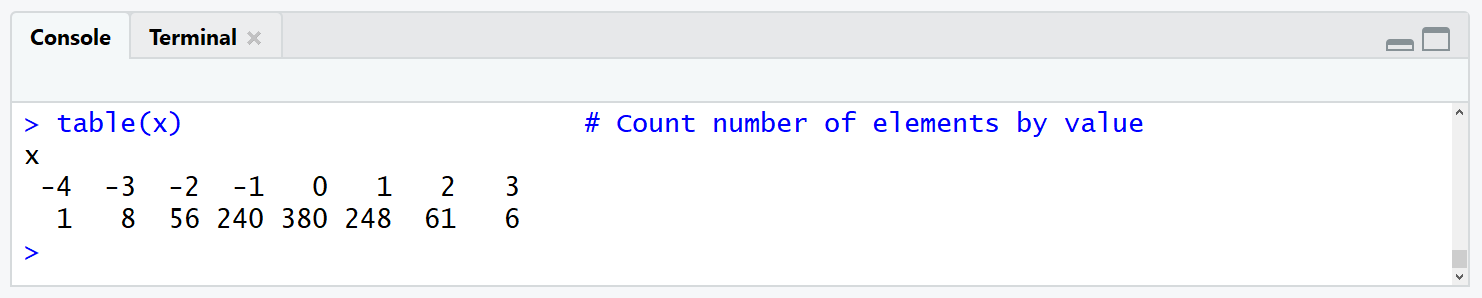# Get Frequency of Elements with Certain Value in Vector (2 R Programming Examples)

In this tutorial, I’ll explain how to count the number of elements with a certain value in the R programming language.

I will show you two programming examples for counting either all elements by their name or only the elements with a given name. The tutorial will contain the following sections:

You’re here for the answer, so let’s get straight to it.

## Data Creation

For the examples of this tutorial, I’m going to use the following data vector:

```set.seed(98765)                  # Set seed
x <- round(rnorm(1000))          # Create vector with random numbers```

Our example vector consists of 1,000 normally distributed numeric values.

## Example 1: Count Number of Elements by their Name

In order to get the frequency of the elements of our data by their name (i.e. by their numeric value), we can use the table() R function. The function can be applied as follows:

`table(x)                         # Count number of elements by value`Figure 1: Frequency Table of Example Vector.

Figure 1 is showing the RStudio console output of the table function. Based on the first line of Figure 1, we can see that our data contains values ranging from -4 to 3. The second row of the output shows the amount how often each of these values appears in our data (e.g. the value -4 occurs only once, the value 0 occurs 380 times, or the value 2 occurs 61 times.

Such tables can be difficult to read. For that reason, I’m showing you how to simplify things in the next example…

## Example 2: Count Number of Elements with Given Name

Let’s assume that we are only interested in the number of elements with a certain value, e.g. the value 2. To extract the number of occurrences of this specific value, we can apply the following R code:

```table(x)[names(table(x)) == 2]   # Count number of elements equal to certain value
#  2
# 61```

The previous R syntax takes a subset of the table that we have created in Example 1. As you can see based on the output of the RStudio console, the value 2 appears 61 times in our data.

## Video & Further Resources

I have published the examples of this R tutorial in a video on my YouTube channel. In case you need some further explanations on how to count the number of times a value appears in a vector or column in R, I can recommend having a look at the video:

Please accept YouTube cookies to play this video. By accepting you will be accessing content from YouTube, a service provided by an external third party.If you accept this notice, your choice will be saved and the page will refresh.

Furthermore, you could have a look at some of the other R tutorials of my homepage. You can find tutorial recommendations below:

In this R programming tutorial you learned how to calculate and count the number of elements with the values of x in a vector, an array, or the column of a data frame. Please let me know in the comments, if you have any further questions.

Subscribe to the Statistics Globe Newsletter